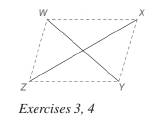Chapter 4.2, Problem 3EElementary Geometry For College St...

7th Edition
Alexander + 2 others
ISBN: 9781337614085

Solutions

Chapter
SectionElementary Geometry For College St...

7th Edition
Alexander + 2 others
ISBN: 9781337614085
Textbook Problem

In the drawing, suppose that W Y ¯ and X Z ¯ bisect each other.What type of quadrilateral is W X Y Z ?To determine

The type of the quadrilateral WXYZ.

Explanation

Given,

WY¯ and XZ¯ are bisected each other.

Still sussing out bartleby?

Check out a sample textbook solution.

See a sample solution

The Solution to Your Study Problems

Bartleby provides explanations to thousands of textbook problems written by our experts, many with advanced degrees!

Get Started

Solve the equations in Exercises 126. (x+1)3+(x+1)5=0

Finite Mathematics and Applied Calculus (MindTap Course List)

True or False: n=1(1)nnn+3 converges.

Study Guide for Stewart's Single Variable Calculus: Early Transcendentals, 8th

True or False: The vector function is continuous at t = 3.

Study Guide for Stewart's Multivariable Calculus, 8th